You are using an outdated browser. Please upgrade your browser to improve your experience.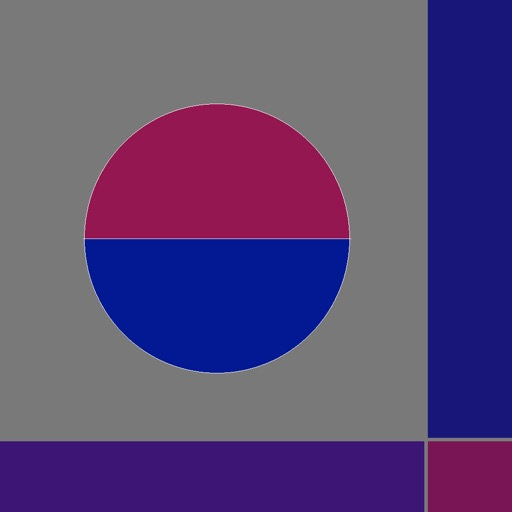## The Semicircle Distribution is the probability distribution supported on the interval [−R, R] the graph of whose probability density function f is a semicircle of radius R centered at (0, 0) and then suitably normalized (so that it is really a semi-ellipse):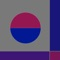# Semicircle Distribution

by Donald Schaefer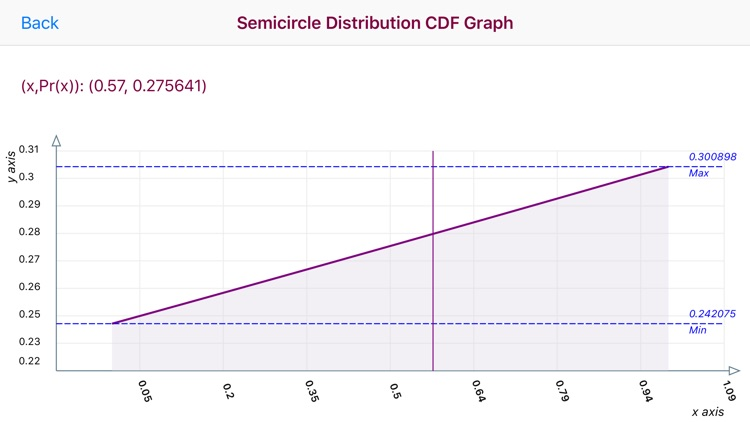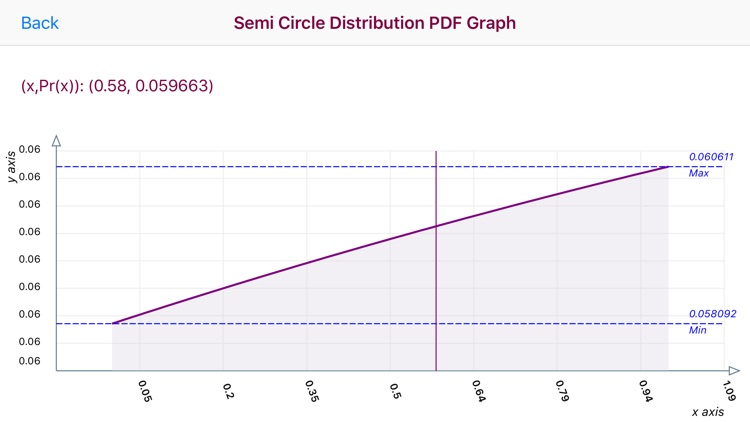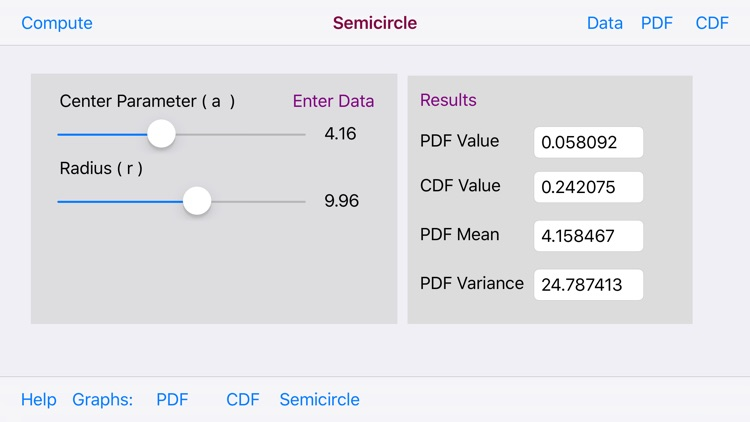### What is it about?

The Semicircle Distribution is the probability distribution supported on the interval [−R, R] the graph of whose probability density function f is a semicircle of radius R centered at (0, 0) and then suitably normalized (so that it is really a semi-ellipse):### App Details

Version
1.1
Rating
NA
Size
7Mb
Genre
Education Productivity
Last updated
January 29, 2019
Release date
September 6, 2016

### App Screenshots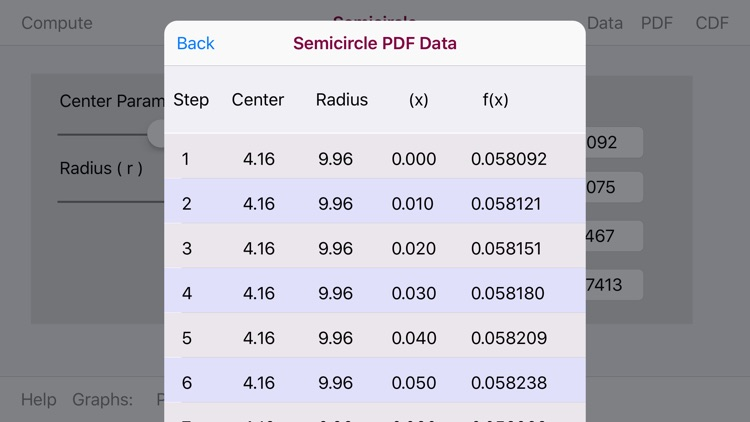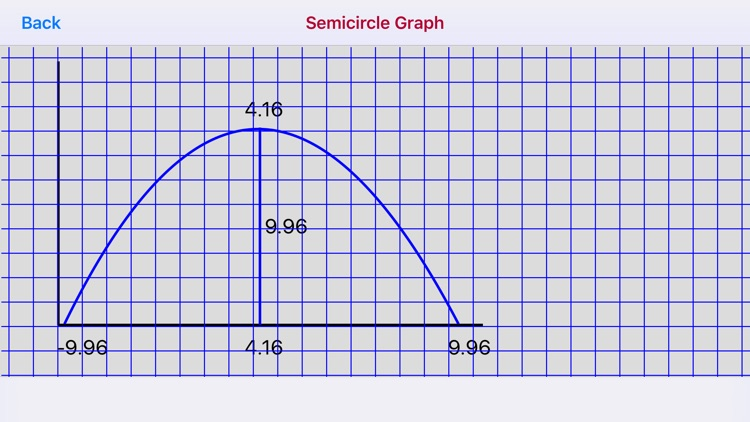### App Store Description

The Semicircle Distribution is the probability distribution supported on the interval [−R, R] the graph of whose probability density function f is a semicircle of radius R centered at (0, 0) and then suitably normalized (so that it is really a semi-ellipse):

Random variable X has the standard semicircle distribution if X has a continuous distribution on [−1,1] with probability density function g given by

f(x;mu,r) = 2*sqrt(r**2 - (x-mu)**2)/(PI*r**2)
mu - r <= x <= mu + r

For the Semicircle Distribution App two data parameters for the Center ( a ) and Radius ( r ) are input via sliders to compute PDF and CDF values and the Semicircle Distribution mean and variance. The PDF and CDF values are displayed both in data table and graph forms.

The PDF and CDF graphs are touch interactive graphs for computed (x/Pr(x) paired values. The graphs have a touch feature whereby upon the touch a slidable vertical line appears. Upon movement of the line a paired (x,Pr(x) values appear relative to the line position on the graph curve.

The horizontal x-axis displays computed (x) values. The vertical y-axis plots a range of Pr(x) values.

Disclaimer:
AppAdvice does not own this application and only provides images and links contained in the iTunes Search API, to help our users find the best apps to download. If you are the developer of this app and would like your information removed, please send a request to [email protected] and your information will be removed.